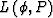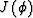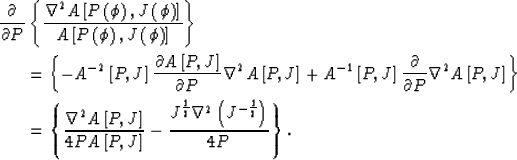Next: MODELING RESULTS Up: SOLVING THE FREQUENCY-DEPENDENT EIKONAL Previous: SOLVING THE FREQUENCY-DEPENDENT EIKONAL

## Evaluation of derivatives of phase slowness with respect to frequency

The first quantity to be evaluated on the right hand side of equation (9) is the ratio between the Laplacian of the amplitude and amplitude itself. This term can be written as(10)

The second term to be evaluated is the derivative ofwith respect to the phase slowness.In evaluating this term I neglect the dependency of the Jacobianon the phase slowness, through the transport equation, because of the difficulties involved in computing the the derivatives of the Jacobian with respect to the phase slowness. Applying the chain rule to equation (10) I obtain:(11)

The exact computation of either (10) or (11) requires the evaluation of the Jacobian at each frequency, and thus it requires performing full dynamic raytracing at each frequency. If results of raytracing were used the extrapolation should be repeated for every source position because the resulting phase-slowness function would be dependent on the source position. In contrast, when the terms dependent on the Jacobian are dropped, the same phase-slowness function can be used for all sources. Further, the Jacobian terms must be evaluated along all the branches of the wavefront. If bifurcations in the wavefront occur, the phase-slowness function would be multivalued. Implementing raytracing through such a function would be more cumbersome than through a single-valued slowness field. On the other hand, dropping the terms dependent on the Jacobian introduces errors where the rate of variations in ray density is large compared with the variations in phase slowness. For example, the accuracy of modeling waves propagating through caustic may be seriously affected.

For computing the results shown in the next section I used only the terms in equations (10) and (11) that are dependent on the phase slowness. As one could have expected, when the contributions of the Jacobian are neglected, the frequency extrapolation results in a progressive smoothing of the slowness function. This smoothing is consistent with the wave equation and, within the limitations in accuracy caused by the approximations that I have introduced, the correct'' amount of smoothing is applied at each frequency.

My raytracing routine does not compute amplitudes yet, thus I could not get a numerical estimate of the errors involved in neglecting the contribution of the Jacobian. However, I experimented with the analytical expression for the Jacobian valid in constant velocity; the inclusion of this homogeneous'' Jacobian had a very small effect on the resulting slowness. I expect that neglecting the contribution of the Jacobian will cause larger errors when the velocity inhomogeneities cause significant variations in ray density. As soon as I complete the implementation of dynamic raytracing on the Connection Machine, I will explore this issue more in depth.

The first term in equation (10) implies that the Laplacian operator is recursively applied to the phase-slowness function. Because the Laplacian operator amplifies the high-wavenumber components of the slowness, its recursive application may cause numerical instability. The high-wavenumber components of the round-off error may grow out of control. Instability can be avoided by applying a band-limited Laplacian. Only the round-off error should be attenuated when the Laplacian is filtered. The cut-off of the low-pass filter applied to the Laplacian must be above the maximum wavenumber present in the slowness function. I also noticed that decreasing the size of the extrapolation step resulted in a more stable solution.Next: MODELING RESULTS Up: SOLVING THE FREQUENCY-DEPENDENT EIKONAL Previous: SOLVING THE FREQUENCY-DEPENDENT EIKONAL
Stanford Exploration Project
11/18/1997